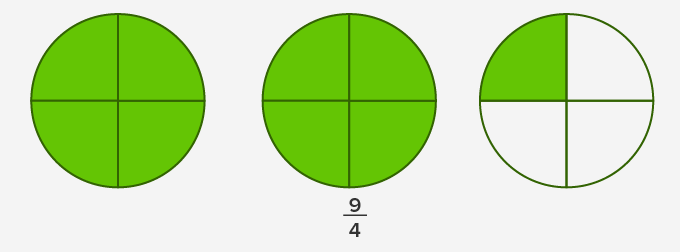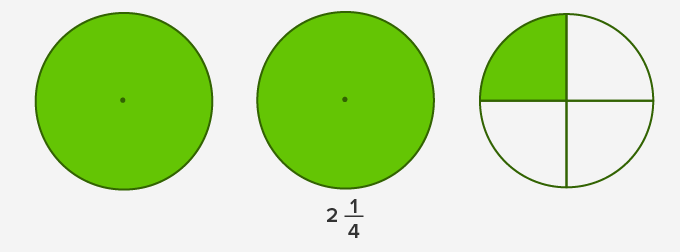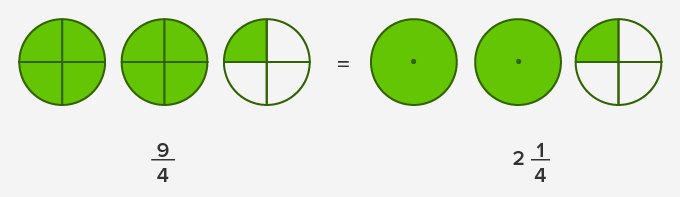Simplify Mixed Numbers - Definition with Examples

The Complete K-5 Math Learning Program Built for Your Child

• 30 Million Kids

Loved by kids and parent worldwide

• 50,000 Schools

Trusted by teachers across schools

• Comprehensive Curriculum

Aligned to Common Core

Simplify Mixed Numbers

Improper fraction

It is a fraction having the numerator (top number) greater than the denominator (bottom number). That’s why at times they are called top-heavy fractions.

Improper fractions represent more than one whole.

For instance:

Example 1: The given picture shows an improper fraction, as there is more than one whole.Mixed numbers

A combined form of a whole number and a fraction is called a mixed number.

So, mixed numbers are more than one whole too.

Example 1: 2 1 4  (two and a quarter) is a mixed number. This can be shown in the following picture.Note:

While reducing a mixed number to the simplest form, we reduce the fraction part and not the whole number.

Improper fractions and mixed fractions are interchangeable. So, we can use either of them to show the same amount.

For instance:

Example 1: In the following picture, 214 = 94 , as shown hereSimplifying mixed numbers

Following are the steps to simplify mixed fractions:

Find the highest common factor (HCF) of numerator and denominator of the fraction part.

Divide both the numerator and the denominator by HCF.

The whole number part will remain the same.

Example 1: Simplify the mixed number  2915 .

Solution: Here, as we need to simplify the fraction part only, so the factors of the numerator are 1, 3, and 9.

Factors of the denominator are 1, 3, 5, and 15.

We can see that 3 is the highest common factor, so we divide the numerator and the denominator by 3.

Therefore,

9 ÷ 3 15 ÷ 3  =  3 5

35 is the simplest form of the fraction part 915 .

Hence, the simplified form of the given mixed number is 235 .

 Fun Facts Converting a mixed number into an improper fraction is just the addition of a whole number and the fraction part together. Example – Convert 235into an improper fraction.r Solution: 235 = 2 + 35               => (21 * 55 ) + 35 = 105 + 35 = 135

Won Numerous Awards & Honors